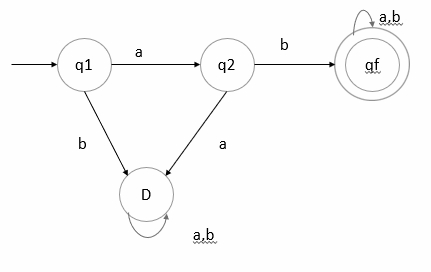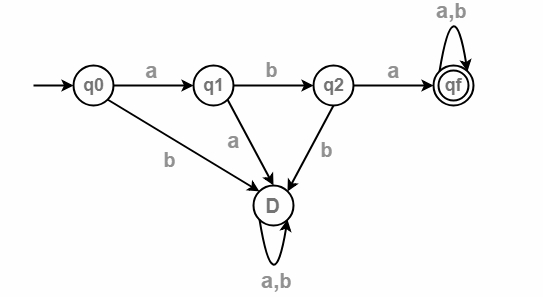# Draw DFA which accepts the string starting with ‘ab’.

## Problem

Draw the state transition diagram over an alphabet Σ={a,b} that accepts the string starting with ‘ab’.

## Solution

The formal definition of Deterministic Finite Automata (DFA) is as follows −

A DFA is a collection of 5-tuples as shown below −

M=(Q, Σ, δ,q0,F)

Where,

• Q: Finite set called states.
• Σ: Finite set called alphabets.
• δ: Q × Σ → Q is the transition function.
• q0 ∈ Q is the initial state.

The language is generated as given below −

L={ab,aba,abab,…….}

The transition diagram is as follows −Here,

• D is a dead state.
• D is a transition state, which it can never escape. Such a state is called a trap state. It is called the dead state.

## Example 1

• ab − q1 on’ a’ goes to q2 and q2 on ‘b’ goes to qf to reach final state. So, ab is accepted.
• baa − q1 on ‘b’ goes to D state, which is dead state. There is no way to reach the final state. So, baa is not accepted.

## Transition table

The transition table is as follows −

State/Input symbolab
qfqfqf
D--

## Explanation

• Step 1 − q1 is an initial state on input a it goes to state q2 and on ‘b’ goes to dead state.
• Step 2 − q2 on ‘a’ goes to dead state and on ‘b’ goes to qf which is the final state.
• Step 3 − qf is the final state on input ‘a’ and ‘b’ goes to qf state itself.
• Step 4 − D is a dead state, there is no path from D to any state.

## Example 2

Design a DFA which accepts a language over the alphabets Σ = {a, b} such that L is the set of all strings starting with ‘aba’.

Therefore, length of substring = 3.

Minimum number of states in the DFA = 3 + 2 = 5.

The language L= {aba,abaa,abaab,abaaba}

The transition diagram is as follows −## Transition table

The transition table is as follows −

State/Input symbolab